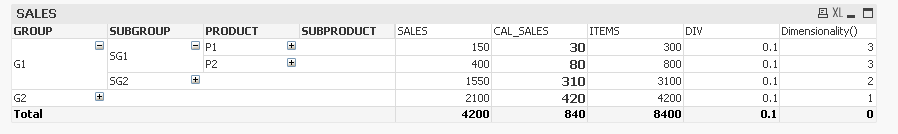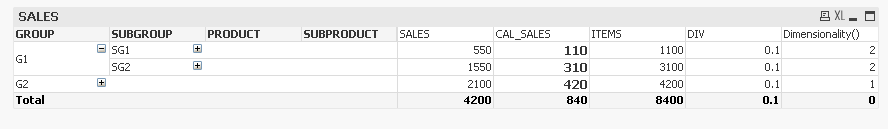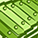# QlikView App Dev

Discussion Board for collaboration related to QlikView App Development.

Announcements
Skip the ticket, Chat with Qlik Support instead for instant assistance.
cancel
Showing results for
Did you mean:Not applicable

## Aggregation is not working

Hi All,

I have 4 dimensions like GROUP, SUBGROUP,PRODUCT,SUBPRODUCT.

Measures SALES,CAL_SALES,ITEMS,DIV

I created a pivot table.CAL_SALES:

For Product P1 SP1,SP2,SP3,SP4,SP5 are the SubProducts.

For Product P2 SP6,SP7,SP8,SP9,SP10 are the SubProducts.

If I minimize Products,Subproducts >>> Need to show Averages of SubProducts,Products.

If I minimize Group,SubGroups >>> Need to show the total of (Averages of SubProducts,Products).

I have used the below expression:

=IF(Dimensionality()=4,AVG(SALES),

IF(Dimensionality()=3,aggr(AVG(SALES),PRODUCT),

IF(Dimensionality()=2,SUM(aggr(AVG(SALES),PRODUCT)),

IF(Dimensionality()=1,SUM(aggr(AVG(SALES),PRODUCT)),

IF(Dimensionality()=0,SUM(aggr(AVG(SALES),PRODUCT,GROUP,SUBGROUP))

)))))

This formula is not working in my real application.

Please check the screens and app.1 Solution

Accepted SolutionsMVP

If you are using conditional show in Pivot Table and only showing one dimension at a time then dimensionality () would be always 1. In that case you don't need such a complex expression.

8 RepliesMVP

I could see the formula is working ..what is the logic your logic for could you explain with an example ?Not applicable
Author

Hi Avinash,

Formula is working in the sample data. But not working in my application.

If I minimize Products,Subproducts >>> Need to show Averages of SubProducts,Products.

If I minimize Group,SubGroups >>> Need to show the total of (Averages of SubProducts,Products).

Could you advise in re-writing the expression?

stalwar1

jagan mohan rao appala

gysbert wassenaar

swuehlMVP

If you mean to say that this formula is working here but not in your real application.. you should provide that sample application or respective data to work.MVP

as per your inputs try this

=IF(Dimensionality()=5,AVG(SALES),

IF(Dimensionality()=4,aggr(AVG(SALES),PRODUCT,SUBPRODUCT),

IF(Dimensionality()=3,(aggr(AVG(SALES),PRODUCT,SUBPRODUCT)),

IF(Dimensionality()=2,SUM(aggr(AVG(SALES),PRODUCT,SUBPRODUCT)),

IF(Dimensionality()=1,SUM(aggr(AVG(SALES),PRODUCT,GROUP,SUBGROUP))

)))))Master

Hi, I dont really get it, but try this one:

=IF(Dimensionality()=4,AVG(SALES),

IF(Dimensionality()=3,avg(aggr(sum(SALES),PRODUCT)),

IF(Dimensionality()=2,avg(aggr(sum(SALES),PRODUCT)),

IF(Dimensionality()=1,avg(aggr(sum(SALES),PRODUCT)),

IF(Dimensionality()=0,avg(aggr(sum(SALES),PRODUCT,GROUP,SUBGROUP))

)))))Partner - Contributor II

Hi,

can you try below one,

IF(Dimensionality()=1, Aggr(Avg(SALES),GROUP),

if(Dimensionality()=2, Aggr(Avg(SALES),SUBGROUP),

if(Dimensionality()=3, Aggr(Avg(SALES),PRODUCT),

if(Dimensionality()=4, Aggr(Avg(SALES),SUBPRODUCT))

)

)

)

if you are using SUM(Aggr(Avg(SALES),SUBGROUP)) also it will give same thing.Contributor III

Hi Gopi,

As per my investigation. This formula is working fine. But, from your words,

"Formula is working in the sample data. But not working in my application". What you mean by this statement ?

Please mention where are you exactly facing the issue with the above solution  ?

Any ways, I am re-writing the expression with few changes,

=IF(Dimensionality()=4,AVG(SALES),

IF(Dimensionality()=3,SUM(aggr(AVG(SALES),PRODUCT)),

IF(Dimensionality()=2,SUM(aggr(AVG(SALES),PRODUCT)),

IF(Dimensionality()=1,SUM(aggr(AVG(SALES),PRODUCT)),

IF(Dimensionality()=0,SUM(aggr(AVG(SALES),PRODUCT,GROUP))

)))))MVP

If you are using conditional show in Pivot Table and only showing one dimension at a time then dimensionality () would be always 1. In that case you don't need such a complex expression.Community Browser